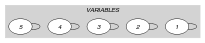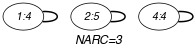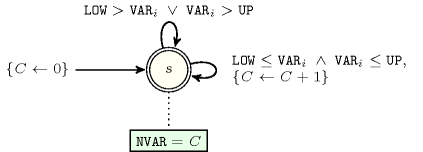## 5.25. among_interval

Origin

Derived from $\mathrm{𝚊𝚖𝚘𝚗𝚐}$.

Constraint

$\mathrm{𝚊𝚖𝚘𝚗𝚐}_\mathrm{𝚒𝚗𝚝𝚎𝚛𝚟𝚊𝚕}\left(\mathrm{𝙽𝚅𝙰𝚁},\mathrm{𝚅𝙰𝚁𝙸𝙰𝙱𝙻𝙴𝚂},\mathrm{𝙻𝙾𝚆},\mathrm{𝚄𝙿}\right)$

Arguments
 $\mathrm{𝙽𝚅𝙰𝚁}$ $\mathrm{𝚍𝚟𝚊𝚛}$ $\mathrm{𝚅𝙰𝚁𝙸𝙰𝙱𝙻𝙴𝚂}$ $\mathrm{𝚌𝚘𝚕𝚕𝚎𝚌𝚝𝚒𝚘𝚗}\left(\mathrm{𝚟𝚊𝚛}-\mathrm{𝚍𝚟𝚊𝚛}\right)$ $\mathrm{𝙻𝙾𝚆}$ $\mathrm{𝚒𝚗𝚝}$ $\mathrm{𝚄𝙿}$ $\mathrm{𝚒𝚗𝚝}$
Restrictions
 $\mathrm{𝙽𝚅𝙰𝚁}\ge 0$ $\mathrm{𝙽𝚅𝙰𝚁}\le |\mathrm{𝚅𝙰𝚁𝙸𝙰𝙱𝙻𝙴𝚂}|$ $\mathrm{𝚛𝚎𝚚𝚞𝚒𝚛𝚎𝚍}$$\left(\mathrm{𝚅𝙰𝚁𝙸𝙰𝙱𝙻𝙴𝚂},\mathrm{𝚟𝚊𝚛}\right)$ $\mathrm{𝙻𝙾𝚆}\le \mathrm{𝚄𝙿}$
Purpose

$\mathrm{𝙽𝚅𝙰𝚁}$ is the number of variables of the collection $\mathrm{𝚅𝙰𝚁𝙸𝙰𝙱𝙻𝙴𝚂}$ taking a value that is located within interval $\left[\mathrm{𝙻𝙾𝚆},\mathrm{𝚄𝙿}$].

Example
$\left(3,〈4,5,8,4,1〉,3,5\right)$

The $\mathrm{𝚊𝚖𝚘𝚗𝚐}_\mathrm{𝚒𝚗𝚝𝚎𝚛𝚟𝚊𝚕}$ constraint holds since we have 3 values, namely $4,5$ and 4 that are situated within interval $\left[3,5\right]$.

Typical
 $\mathrm{𝙽𝚅𝙰𝚁}>0$ $\mathrm{𝙽𝚅𝙰𝚁}<|\mathrm{𝚅𝙰𝚁𝙸𝙰𝙱𝙻𝙴𝚂}|$ $|\mathrm{𝚅𝙰𝚁𝙸𝙰𝙱𝙻𝙴𝚂}|>1$ $\mathrm{𝙻𝙾𝚆}<\mathrm{𝚄𝙿}$ $\mathrm{𝙻𝙾𝚆}\le$$\mathrm{𝚖𝚊𝚡𝚟𝚊𝚕}$$\left(\mathrm{𝚅𝙰𝚁𝙸𝙰𝙱𝙻𝙴𝚂}.\mathrm{𝚟𝚊𝚛}\right)$ $\mathrm{𝚄𝙿}\ge$$\mathrm{𝚖𝚒𝚗𝚟𝚊𝚕}$$\left(\mathrm{𝚅𝙰𝚁𝙸𝙰𝙱𝙻𝙴𝚂}.\mathrm{𝚟𝚊𝚛}\right)$
Symmetries
• Items of $\mathrm{𝚅𝙰𝚁𝙸𝙰𝙱𝙻𝙴𝚂}$ are permutable.

• An occurrence of a value of $\mathrm{𝚅𝙰𝚁𝙸𝙰𝙱𝙻𝙴𝚂}.\mathrm{𝚟𝚊𝚛}$ that belongs to $\left[\mathrm{𝙻𝙾𝚆},\mathrm{𝚄𝙿}\right]$ (resp. does not belong to $\left[\mathrm{𝙻𝙾𝚆},\mathrm{𝚄𝙿}\right]$) can be replaced by any other value in $\left[\mathrm{𝙻𝙾𝚆},\mathrm{𝚄𝙿}\right]$) (resp. not in $\left[\mathrm{𝙻𝙾𝚆},\mathrm{𝚄𝙿}\right]$).

Arg. properties
• Functional dependency: $\mathrm{𝙽𝚅𝙰𝚁}$ determined by $\mathrm{𝚅𝙰𝚁𝙸𝙰𝙱𝙻𝙴𝚂}$, $\mathrm{𝙻𝙾𝚆}$ and $\mathrm{𝚄𝙿}$.

• Contractible wrt. $\mathrm{𝚅𝙰𝚁𝙸𝙰𝙱𝙻𝙴𝚂}$ when $\mathrm{𝙽𝚅𝙰𝚁}=0$.

• Contractible wrt. $\mathrm{𝚅𝙰𝚁𝙸𝙰𝙱𝙻𝙴𝚂}$ when $\mathrm{𝙽𝚅𝙰𝚁}=|\mathrm{𝚅𝙰𝚁𝙸𝙰𝙱𝙻𝙴𝚂}|$.

• Aggregate: $\mathrm{𝙽𝚅𝙰𝚁}\left(+\right)$, $\mathrm{𝚅𝙰𝚁𝙸𝙰𝙱𝙻𝙴𝚂}\left(\mathrm{𝚞𝚗𝚒𝚘𝚗}\right)$, $\mathrm{𝙻𝙾𝚆}\left(\mathrm{𝚒𝚍}\right)$, $\mathrm{𝚄𝙿}\left(\mathrm{𝚒𝚍}\right)$.

Remark

By giving explicitly all values of the interval $\left[\mathrm{𝙻𝙾𝚆},\mathrm{𝚄𝙿}\right]$ the $\mathrm{𝚊𝚖𝚘𝚗𝚐}_\mathrm{𝚒𝚗𝚝𝚎𝚛𝚟𝚊𝚕}$ constraint can be modelled with the $\mathrm{𝚊𝚖𝚘𝚗𝚐}$ constraint. However when $\mathrm{𝙻𝙾𝚆}-\mathrm{𝚄𝙿}+1$ is a large quantity the $\mathrm{𝚊𝚖𝚘𝚗𝚐}_\mathrm{𝚒𝚗𝚝𝚎𝚛𝚟𝚊𝚕}$ constraint provides a more compact form.

generalisation: $\mathrm{𝚊𝚖𝚘𝚗𝚐}$ ($\mathrm{𝚟𝚊𝚛𝚒𝚊𝚋𝚕𝚎}$ in $\mathrm{𝚒𝚗𝚝𝚎𝚛𝚟𝚊𝚕}$ replaced by $\mathrm{𝚟𝚊𝚛𝚒𝚊𝚋𝚕𝚎}\in \mathrm{𝚟𝚊𝚕𝚞𝚎𝚜}$).

Keywords
Arc input(s)

$\mathrm{𝚅𝙰𝚁𝙸𝙰𝙱𝙻𝙴𝚂}$

Arc generator
$\mathrm{𝑆𝐸𝐿𝐹}$$↦\mathrm{𝚌𝚘𝚕𝚕𝚎𝚌𝚝𝚒𝚘𝚗}\left(\mathrm{𝚟𝚊𝚛𝚒𝚊𝚋𝚕𝚎𝚜}\right)$

Arc arity
Arc constraint(s)
 $•\mathrm{𝙻𝙾𝚆}\le \mathrm{𝚟𝚊𝚛𝚒𝚊𝚋𝚕𝚎𝚜}.\mathrm{𝚟𝚊𝚛}$ $•\mathrm{𝚟𝚊𝚛𝚒𝚊𝚋𝚕𝚎𝚜}.\mathrm{𝚟𝚊𝚛}\le \mathrm{𝚄𝙿}$
Graph property(ies)
$\mathrm{𝐍𝐀𝐑𝐂}$$=\mathrm{𝙽𝚅𝙰𝚁}$

Graph model

The arc constraint corresponds to a unary constraint. For this reason we employ the $\mathrm{𝑆𝐸𝐿𝐹}$ arc generator in order to produce a graph with a single loop on each vertex.

Parts (A) and (B) of Figure 5.25.1 respectively show the initial and final graph associated with the Example slot. Since we use the $\mathrm{𝐍𝐀𝐑𝐂}$ graph property, the loops of the final graph are stressed in bold.

##### Figure 5.25.1. Initial and final graph of the $\mathrm{𝚊𝚖𝚘𝚗𝚐}_\mathrm{𝚒𝚗𝚝𝚎𝚛𝚟𝚊𝚕}$ constraint(a) (b)
Automaton

Figure 5.25.2 depicts the automaton associated with the $\mathrm{𝚊𝚖𝚘𝚗𝚐}_\mathrm{𝚒𝚗𝚝𝚎𝚛𝚟𝚊𝚕}$ constraint. To each variable ${\mathrm{𝚅𝙰𝚁}}_{i}$ of the collection $\mathrm{𝚅𝙰𝚁𝙸𝙰𝙱𝙻𝙴𝚂}$ corresponds a 0-1 signature variable ${S}_{i}$. The following signature constraint links ${\mathrm{𝚅𝙰𝚁}}_{i}$ and ${S}_{i}$: $\mathrm{𝙻𝙾𝚆}\le {\mathrm{𝚅𝙰𝚁}}_{i}\wedge {\mathrm{𝚅𝙰𝚁}}_{i}\le \mathrm{𝚄𝙿}⇔{S}_{i}$. The automaton counts the number of variables of the $\mathrm{𝚅𝙰𝚁𝙸𝙰𝙱𝙻𝙴𝚂}$ collection that take their value in $\left[\mathrm{𝙻𝙾𝚆},\mathrm{𝚄𝙿}$] and finally assigns this number to $\mathrm{𝙽𝚅𝙰𝚁}$.

##### Figure 5.25.2. Automaton of the $\mathrm{𝚊𝚖𝚘𝚗𝚐}_\mathrm{𝚒𝚗𝚝𝚎𝚛𝚟𝚊𝚕}$ constraint##### Figure 5.25.3. Hypergraph of the reformulation corresponding to the automaton (with one counter) of the $\mathrm{𝚊𝚖𝚘𝚗𝚐}_\mathrm{𝚒𝚗𝚝𝚎𝚛𝚟𝚊𝚕}$ constraint: since all states variables ${Q}_{0},{Q}_{1},\cdots ,{Q}_{n}$ are fixed to the unique state $s$ of the automaton, the transitions constraints share only the counter variable $C$ and the constraint network is Berge-acyclic